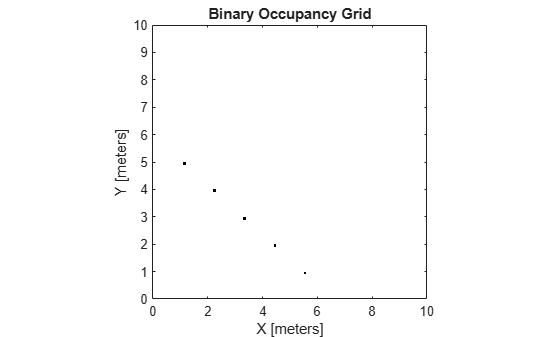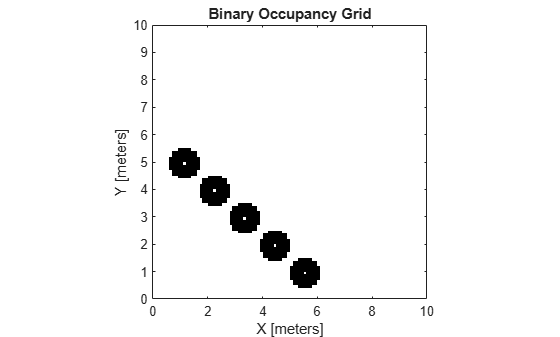# world2grid

Convert world coordinates to grid indices

## Syntax

``ij = world2grid(map,xy)``

## Description

example

````ij = world2grid(map,xy)` converts an array of world coordinates, `xy`, to a ```[rows cols]``` array of grid indices, `ij`.```

## Examples

collapse all

Create an empty binary occupancy map with a width and height of 10 meters.

`map = binaryOccupancyMap(10,10);`

Get grid indices from world coordinates.

```[xWorld,yWorld] = meshgrid(0:0.5:2); ij = world2grid(map,[xWorld(:) yWorld(:)]);```

Create a 10m x 10m empty map.

`map = binaryOccupancyMap(10,10,10);`

Set occupancy of world locations and show map.

```x = [1.2; 2.3; 3.4; 4.5; 5.6]; y = [5.0; 4.0; 3.0; 2.0; 1.0]; setOccupancy(map, [x y], ones(5,1)) figure show(map)```Inflate occupied locations by a given radius.

```inflate(map, 0.5) figure show(map)```Get grid locations from world locations.

`ij = world2grid(map, [x y]);`

Set grid locations to free locations.

```setOccupancy(map, ij, zeros(5,1), 'grid') figure show(map)```## Input Arguments

collapse all

Map representation, specified as a `binaryOccupancyMap` object.

World coordinates, specified as an n-by-2 vertical array of ```[x y]``` pairs, where n is the number of world coordinates.

## Output Arguments

collapse all

Grid indices, specified as an n-by-2 vertical array of `[i j]` pairs in `[rows cols]` format, where n is the number of grid positions.

## Version History

Introduced in R2015a